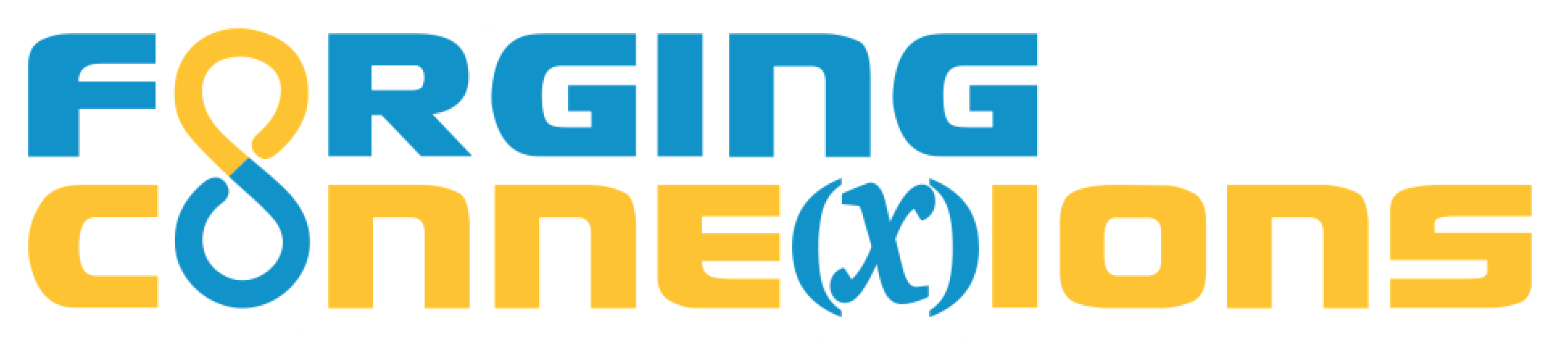This site supports the NSF project on Technology-Rich Units for Future Secondary Teachers: Forging Dynamic Connections Between Geometry and Functions, which is part of the NSF's program on Improving Undergraduate STEM Education (IUSE).

Unit 2: Experiment with Composition and Congruence

1. Mystery Transformations: The student chooses a triangle transformation tool and adjusts the transformation to superpose one triangle on another. Should this also include a sketch in which the student chooses a point transformation tool and matches one shape polygon to another by restricting the independent variable to the polygonal domain?
2. Mirror, Mirror: The student uses the Reflect tool and adjusts the result to superpose one triangle on another. The five pages of the sketch include triangles that have been reflected, rotated, dilated, translated, and glide-reflected. The sketch includes the tools needed to construct mirrors for each problem instead of adjusting them. Should this web page include two sketches, one with only the Reflect tool for students to adjust, and one with the additional tools asking students to construct the necessary mirror?
3. The Segment Congruence Theorem: The student first adjusts one or two mirrors to superpose the segments, and then constructs the mirrors that put the segments into superposition. As an extension, students are challenged to construct a single rotation that superposes two general segments, and then a single glide reflection that superposes two general segments.
4. The Side-Side-Side Theorem: The student's first task is to use SSS to construct triangles: given three segments of various lengths, construct a triangle with side lengths that match the given segments, clearly establishing what we mean by SSS. In the second task, the student constructs one, two, or three mirrors to superpose two triangles that have their three sides related by SSS. The four pages of the sketch have four possible cases (corresponding to reflection, translation, rotation, and glide reflection). In a third optional task, the student is challenged to construct a single transformation for each case.
5. Explore Similarity: In the first activity students adjust some combination of dilation and reflection to superpose one triangle on another. In the second activity, students dilate triangles and investigate the similarities and differences between the pre-image triangle and its image.
6. SAS, SSA, and ASA: (Not yet available) Students work either sequentially or in small groups to solve three tasks, each of the following form: Are triangles connected by ___ always congruent? If so, prove it by construction; if not, construct a counter-example. Fill in the blank with SAS, SSA, or ASA to describe each task.